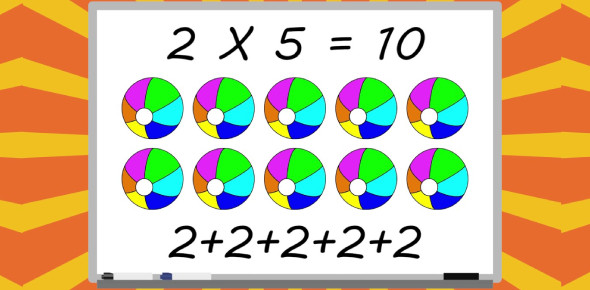# Mathematics Quiz On Basic Multiplication For Kids!

18 Questions | Attempts: 6177SettingsThis is a Mathematics Quiz on Basic Multiplication for Kids! When it comes to multiplying figures, be they large or small most people have a hard time doing the calculations without the use of calculators. Do you need some help solving some mathematical problems? Let us see if we can help you out by you trying out this test below and seeing if you can find the answer what happens when you multiply a number by six.

• 1.
6*8=
• A.

48

• B.

12

• C.

68

• 2.
6*2=
• A.

8

• B.

62

• C.

12

• 3.
6*6=
• A.

66

• B.

36

• C.

12

• 4.
6*9=
• A.

37

• B.

26

• C.

54

• 5.
6*7=
• A.

47

• B.

42

• C.

48

• 6.
6*5=
• A.

30

• B.

35

• C.

25

• 7.
6*3=
• A.

24

• B.

81

• C.

18

• 8.
6*1=
• A.

12

• B.

6

• C.

78

• 9.
6*4=
• A.

24

• B.

42

• C.

12

• 10.
6*11=
• A.

65

• B.

67

• C.

66

• 11.
6*10=
• A.

60

• B.

4

• C.

71

• 12.
6*12=
• A.

64

• B.

2

• C.

72

• 13.
6*8=
• A.

43

• B.

48

• C.

81

• 14.
6*2=
• A.

42

• B.

21

• C.

12

• 15.
6*9=
• A.

45

• B.

54

• C.

66

• 16.
6*7=
• A.

42

• B.

69

• C.

65

• 17.
6*11=
• A.

55

• B.

77

• C.

66

• 18.
6*12=
• A.

72

• B.

27

• C.

34

## Related TopicsBack to top
×

Wait!
Here's an interesting quiz for you.